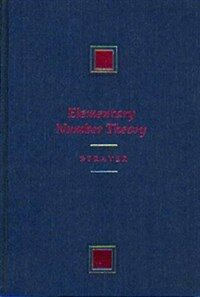> 상세정보

# 상세정보## Elementary number theory (2회 대출)

자료유형
단행본
개인저자
Strayer, James K.
서명 / 저자사항
Elementary number theory / James K. Strayer.
발행사항
Boston :   PWS Pub. Co.,   c1994.
형태사항
xiv, 290 p. : ill. ; 25 cm.
ISBN
0534936725 (acid-free, recycled paper)
서지주기
Includes bibliographical references (p. 284-286) and index.
일반주제명
Number theory.
 000 00704camuuu200241 a 4500 001 000000424827 003 OCoLC 005 19960729121739.0 008 930317s1994 maua b 001 0 eng 010 ▼a 93017125 020 ▼a 0534936725 (acid-free, recycled paper) 040 ▼a DLC ▼c DLC 049 ▼a OCLC ▼l 111049988 050 0 0 ▼a QA241 ▼b .S817 1994 082 0 0 ▼a 512/.72 ▼2 20 090 ▼a 512.72 ▼b S913e 100 1 ▼a Strayer, James K. 245 1 0 ▼a Elementary number theory / ▼c James K. Strayer. 260 ▼a Boston : ▼b PWS Pub. Co., ▼c c1994. 300 ▼a xiv, 290 p. : ▼b ill. ; ▼c 25 cm. 504 ▼a Includes bibliographical references (p. 284-286) and index. 650 0 ▼a Number theory.

### 소장정보

No. 소장처 청구기호 등록번호 도서상태 반납예정일 예약 서비스
No. 1 소장처 청구기호 512.72 S913e 등록번호 111049988 도서상태 대출가능 반납예정일 예약 서비스

### 컨텐츠정보

#### 목차

```CONTENTS
Introduction = 1
1 Divisibility and Factorization = 3
1.1 Divisibility = 3
1.2 Prime Numbers = 10
1.3 Greatest Common Divisors = 18
1.4 The Euclidean Algorithm = 22
1.5 The Fundamental Theorem of Arithmetic = 26
1.6 Concluding Remarks = 36
Student Projects = 36
2 Congruences = 38
2.1 Congruences = 38
2.2 Linear Congruences in One Variable = 48
2.3 The Chinese Remainder Theorem = 54
2.4 Wilson's Theorem = 59
2.5 Fermat's Little Theorem: Pseudoprime Numbers = 63
2.6 Euler's Theorem = 68
2.7 Concluding Remarks = 73
Student Projects = 73
3 Arithmetic Functions = 76
3.1 Arithmetic Functions: Multiplicativity = 76
3.2 The Euler Phi-Function = 81
3.3 The Number of Positive Divisors Function = 86
3.4 The Sum of Positive Divisors Function = 89
3.5 Perfect Numbers = 92
3.6 The M o ·· bius Inversion Formula = 96
3.7 Concluding Remarks = 101
Student Projects = 101
4.2 The Legendre Symbol = 108
4.3 The Law of Quadratic Reciprocity = 117
4.4 Concluding Remarks = 126
Student Projects = 126
5 Primitive Roots = 128
5.1 The Order of an Integer; Primitive Roots = 128
5.2 Primitive Roots for Prime Numbers = 134
5.3 The Primitive Root Theorem = 140
5.4 Index Arithmetic: nth Power Residues = 146
5.5 Concluding Remarks = 152
Student Projects = 152
6 Diophantine Equations = 154
6.1 Linear Diophantine Equations = 154
6.2 Nonlinear Diophantine Equations; a Congruence Method = 159
6.3 Pythagorean Triples = 161
6.4 Fermat's Last Theorem = 164
6.5 Representation of an Integer as a Sum of Squares = 168
6.6 Concluding Remarks = 179
Student Projects = 180
7 Continued Fractions = 181
7.1 Rational and Irrational Numbers = 181
7.2 Finite Continued Fractions = 190
7.3 Convergents = 195
7.4 Infinite Continued Fractions = 202
7.5 Eventually Periodic Continued Fractions = 214
7.6 Periodic Continued Fractions = 223
7.7 Concluding Remarks = 228
Student Projects = 229
8 A Few Applications = 231
8.1 A Recreational Application = 231
8.2 Cryptography: The RSA Encryption System = 233
8.3 Primality Testing = 239
8.4 Pell's Equation = 241
8.5 Concluding Remarks = 248
Student Projects = 249
Appendices = 251
A Mathematical Induction = 253
B Equivalence Relations = 257
C Abstract Algebra = 261
D The Binomial Theorem = 264
E Tables = 266
Hints ana Answers to Selected Exercises = 272
Bibliography = 284
Index = 287```